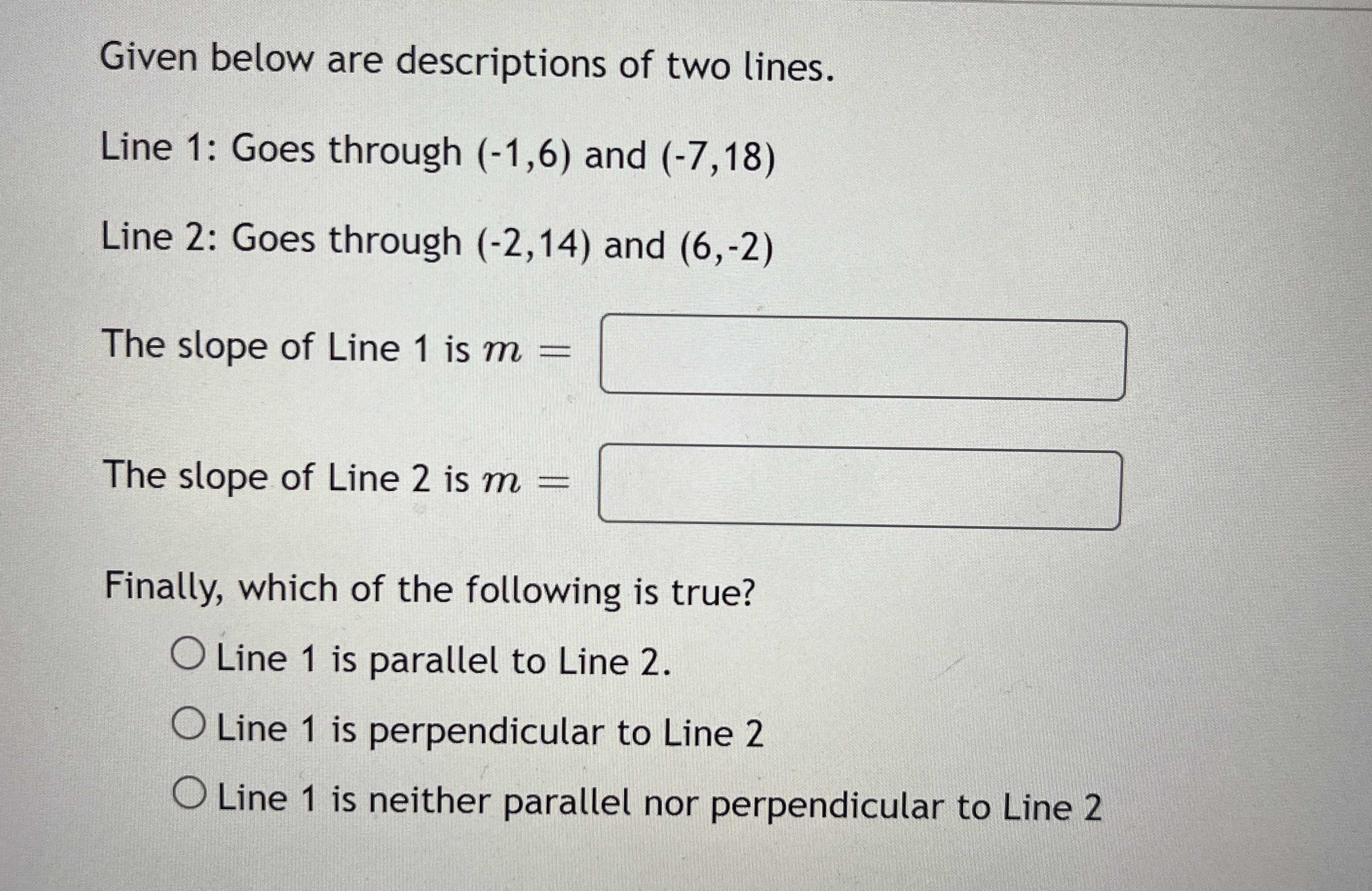### ¿Todavía tienes preguntas de matemáticas?

Pregunte a nuestros tutores expertos
Algebra
PreguntaGiven below are descriptions of two lines. Line 1: Goes through $$( - 1,6 )$$ and $$( - 7,18 )$$

Line 2: Goes through $$( - 2,14 )$$ and $$( 6 , - 2 )$$

The slope of Line $$1$$ is $$m =$$ The slope of Line $$2$$ is $$m =$$

Finally, which of the following is true? Line $$1$$ is parallel to Line $$2$$ . Line $$1$$ is perpendicular to Line $$2$$ Line $$1$$ is neither parallel nor perpendicular to Line $$2$$